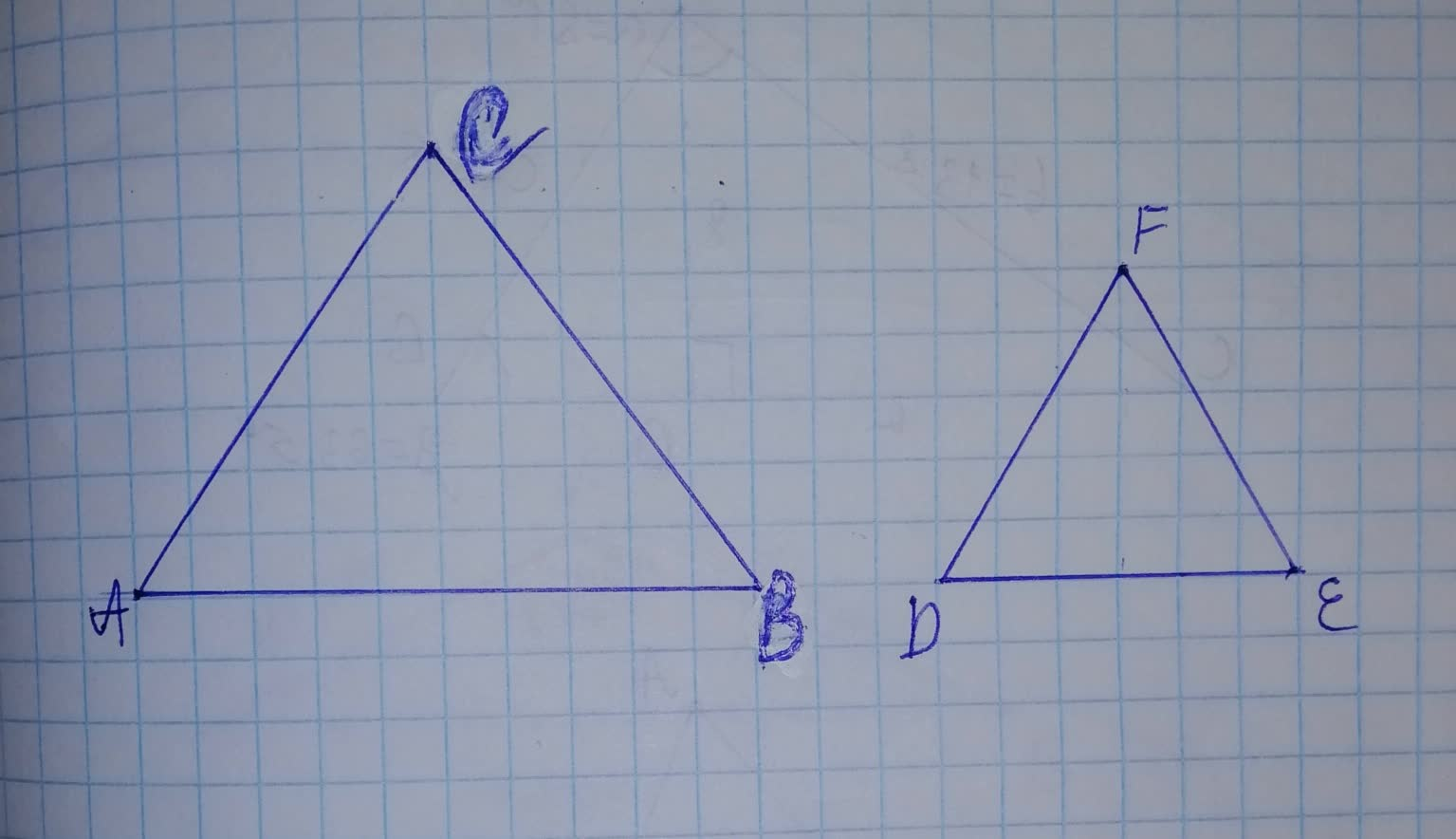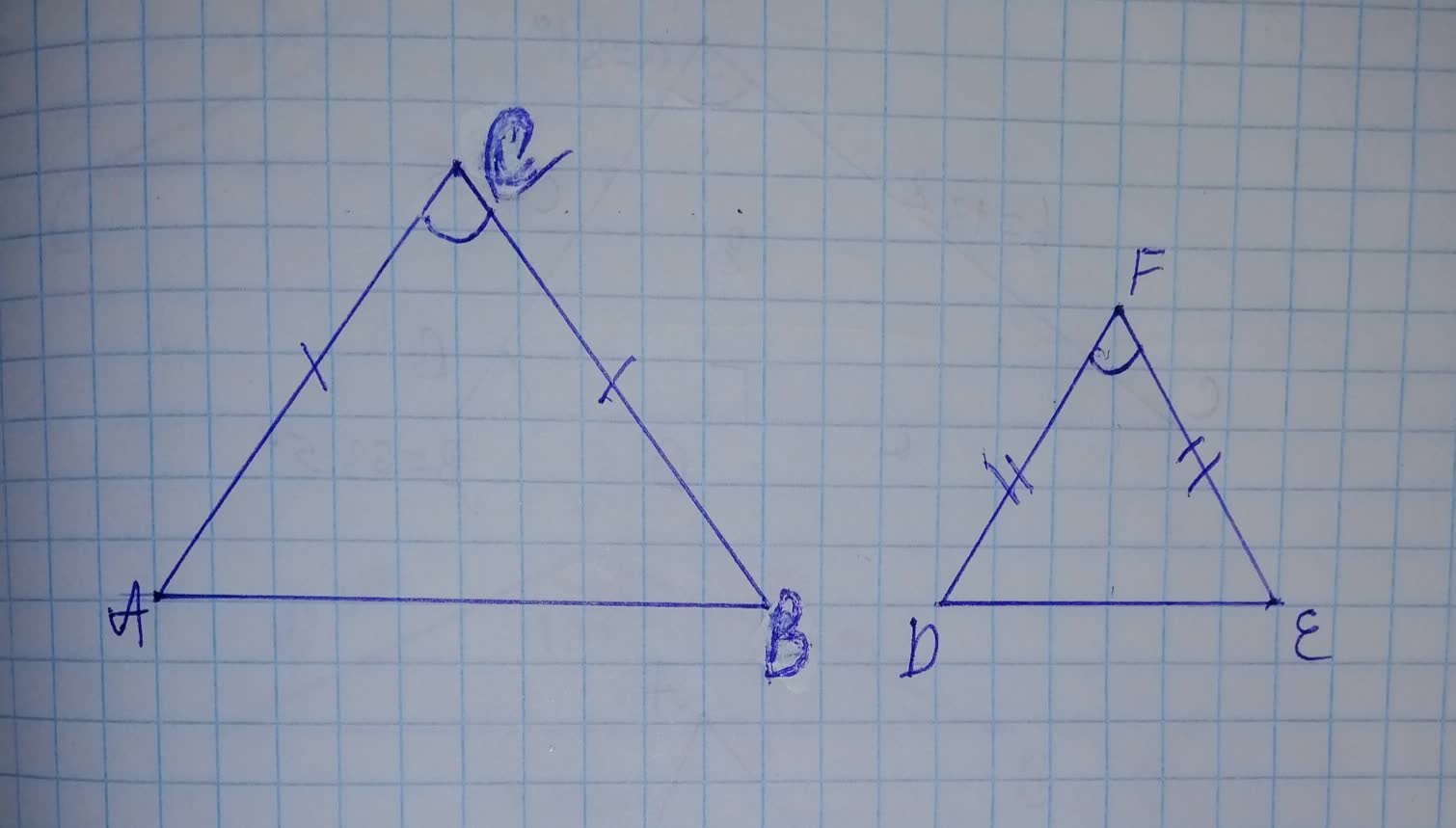Prove that two isosceles triangles are similar if any angle of one equals the coBarbara Schroder 2021-11-21 Answered

Prove that two isosceles triangles are similar if any angle of one equals the corresponding angle of the other.a. Case 1: Equal Vertex Angles
Given Isosceles $$\displaystyle\triangle{A}{B}{C}$$, isosceles $$\displaystyle\triangle{D}{E}{F},\ {A}{C}={B}{C},\ {D}{F}={E}{F},\ \angle{C}=\angle{F}$$
Prove: $$\displaystyle\triangle{A}{B}{C}\sim\triangle{D}{E}{F}$$
$\begin{array}{|c|c|}\hline \text{Statements} & \text{Reasons} \\ \hline 1. (see\ above) & \text{1. Given} \\ \hline 2. & \text{2. Isosceles triangles with equal vertex} \\ & \text{angles have equal base angles also.} \\ \hline 3. \triangle ABC\sim\triangle DEF & \text{3. } \\ \hline \end{array}$

• Questions are typically answered in as fast as 30 minutes

Solve your problem for the price of one coffee

• Math expert for every subject
• Pay only if we can solve itDrood1980
Step 1
Consider the figure:$$\displaystyle\angle{A}{C}{B}=\angle{D}{F}{E}$$
$$\displaystyle{A}{C}={B}{C}$$
$$\displaystyle{D}{F}={E}{F}$$
Step 2
Find the statement for isosceles triangles with equal vertex angles have equal base angles.
Given:
$$\displaystyle\angle{A}{C}{B}=\angle{D}{F}{E}$$
$$\displaystyle{A}{C}={B}{C}$$
$$\displaystyle{D}{F}={E}{F}$$
If two isosceles triangles with equal vertex angles, then they have equal base angles also.
Since, $$\displaystyle\angle{A}{C}{B}=\angle{D}{F}{E}$$
$$\displaystyle\angle{C}{A}{B}=\angle{F}{D}{E}$$
and,
$$\displaystyle\angle{C}{B}{A}=\angle{F}{E}{D}$$
$\begin{array}{|c|c|}\hline 2.\angle CAB=\angle FDE \\ \angle CBA=\angle FED & \text{2. Isosceles triangles with equal vertex} \\ & \text{angles have equal base angles also.} \\ \hline \end{array}$
Step 3
Find the reason of similarity of given triangles.
Use theorem 57, If two triangles have the three angles of one equal respectively to the three angles of the other, then the triangles are similar.
Corollary 57-1 If two angles of one triangle are equal respectively to two angles of another, then the triangles are similar. (a.a)
Theorem 58-If two triangles have two pairs of sides proportional and the included angles equal respectively, then the two triangles are similar. (s.a.s)
$$\displaystyle\angle{A}{C}{B}=\angle{D}{F}{E}$$ (Theorem 57)
$$\displaystyle\angle{C}{A}{B}=\angle{F}{D}{E}$$ and $$\displaystyle\angle{C}{B}{A}=\angle{F}{E}{D}$$ (Corollary 57-1)
$$\displaystyle{\frac{{{A}{C}}}{{{D}{F}}}}={\frac{{{B}{C}}}{{{E}{F}}}}$$ and All above equal angles (Theorem 58)
So, $$\displaystyle\triangle{A}{B}{C}=\triangle{D}{E}{F}$$
$\begin{array}{|c|c|}\hline 3.\triangle ABC=\triangle DEF & \text{3. If two triangles have three angles} \\ & \text{of one equal respectively to the} \\ & \text{three angles of the other, then} \\ & \text{the triangles are similar.} \\ \hline \end{array}$